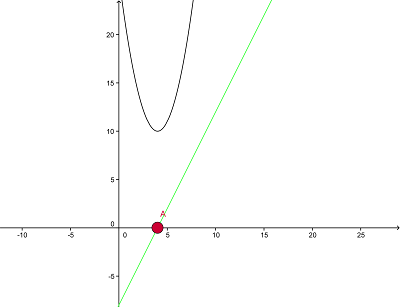Feb 27, 2012

Nonlinear conjugate gradient method in Java

Hi all,

I have been a bit busy for the last few weeks with some projects. But fortunately I found some time on the last weekend to implement a recommandation engine for myself based on what we have done in ml-class.org lessons in octave and translate it to a Java version.

If you attended ml-class you might be familiar that you need to minimize a rather complex cost function based on what the user likes in terms of movies.
However I haven't found a simple and not ancient Java library containing a fully working conjugate gradient method. Shorthand I decided to translate it from Octave to Java. It took me 5-6 hours to build a Octave-like vector library arround it to translate it quite 1:1. But it was really worth it.

And here it is:
https://github.com/thomasjungblut/thomasjungblut-common/blob/master/src/de/jungblut/math/minimize/Fmincg.java

Fmincg btw stands for Function minimize nonlinear conjugant gradient. It wasn't clear for me in the first place and I really started to understand the algorithm when I translated it.

It works quite like the version in octave, you pass an input vector (which is used as a starting point) and a costfunction along with a number of iterations to do.
Since I'm a hacker by heart, I want to give you a sample code to try it out for yourself.

Example

I have prepared a simple quadratic function for you
f(x) = (4-x)^2+10

Obviously since this is quadratic this has a global minimum which is easy to spot because I used the binomial version of the function, we will see if fmincg finds it.

For the algorithm we constantly need to calculate the gradients of the input in our cost function. Therefore we need the derivative which is for our function
f(x)' = 2x-8

If you're a math crack then you know that the f(x) hits (int our case) the minimum where the derivative cut's the x-axis or y=0.

Since this is quite hard to visualize, I have made a picture for you:Function f(x) and its derivative
Not difficult to spot, you see the black function is our f(x) whereas the green line is our derivative. And it hits the x-axis right at the minimum of the function. Great!

How do we code this?

This is quite simple, I show you the code first:

int startPoint = -5;
// start at x=-5
DenseDoubleVector start = new DenseDoubleVector(new double[] { startPoint });

CostFunction inlineFunction = new CostFunction() {
@Override
public Tuple<Double, DenseDoubleVector> evaluateCost(
DenseDoubleVector input) {

// our function is f(x) = (4-x)^2+10, so we can calculate the cost
double cost = Math.pow(4-input.get(0),2)+10;
// the derivative is f(x)' = 2x-8 which is our gradient value
DenseDoubleVector gradient = new DenseDoubleVector(new double[] {2*input.get(0)-8});

return new Tuple<Double, DenseDoubleVector>(cost, gradient);
}
};

DenseDoubleVector minimizeFunction = Fmincg.minimizeFunction(inlineFunction, start, 100, true);
// should return 4
System.out.println("Found a minimum at: " + minimizeFunction);

As you can see we have to allocate the vector which is containing our start "point". You can set this arbitrary randomly, but you have know that it might not hit the global minimum but rather a local minimum. So different random starting points can yield to different results.

But not in our case. Now you can implement the cost function the algorithm needs the cost of your function at the given point of the input and the value of the derivative at this point.
So we return this after we put the input "x" in our two equations as a tuple.

The whole application prints:

Interation 1 | Cost: 10,000000
Interation 2 | Cost: 10,000000
[4.0]

Works! And it outputs the x-value of our minima and the cost is 10, which should be the y-value. Very cool!
You can find this example on Github as well.

Please use it if you need it, I have already implemented the collaborative filtering algorithm with it and I guess a backprop neural network will follow soon.

I really have to admit that I am not a math crack although I am studying computer sciences, but math really makes many things easier and if you take a deeper look behind what you've learned in school, it is really beautiful.

Thanks and bye!